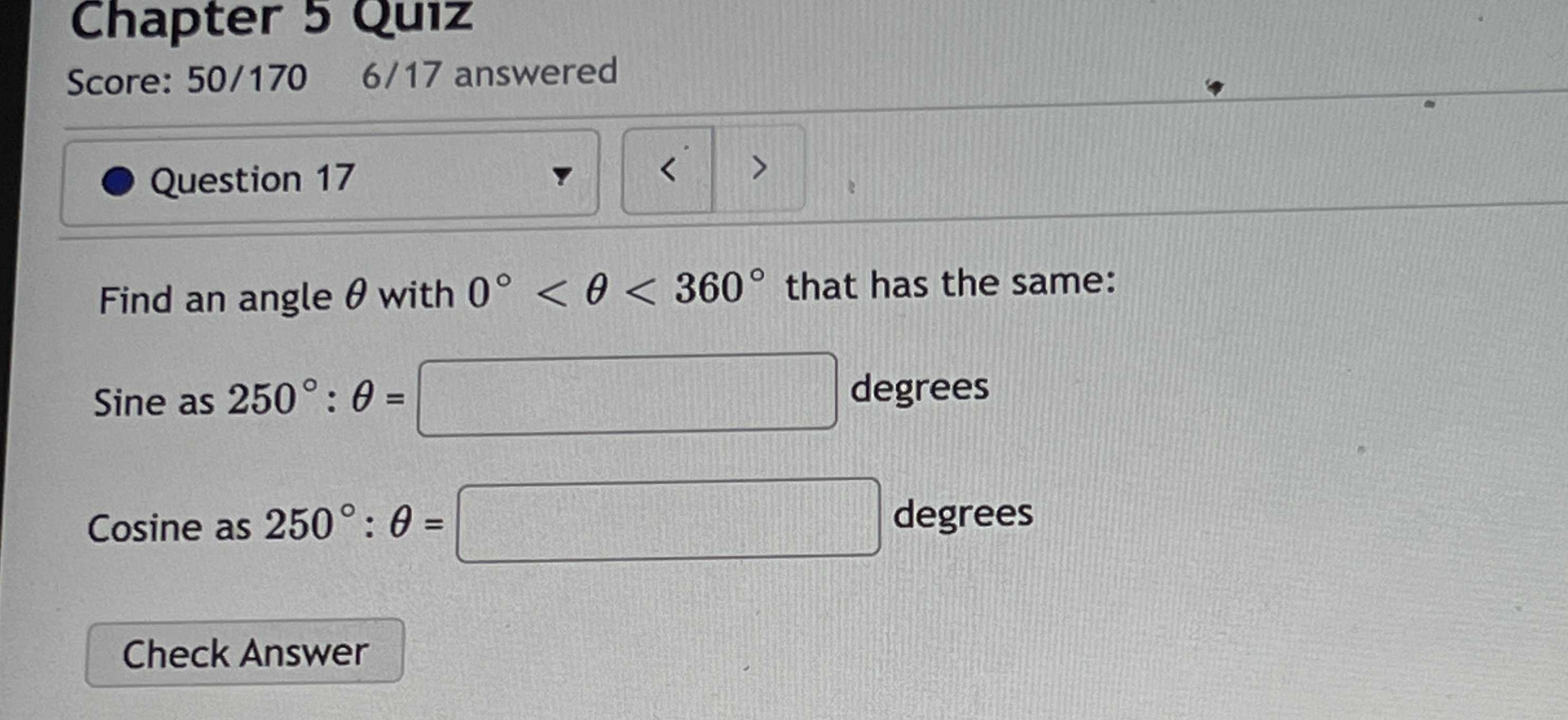### Still have math questions?

Trigonometry
QuestionFind an angle $$\theta$$ with $$0 ^ { \circ } < \theta < 360 ^ { \circ }$$ that has the same: Sine as $$250 ^ { \circ } : \theta =$$ degrees Cosine as $$250 ^ { \circ } : \theta =$$